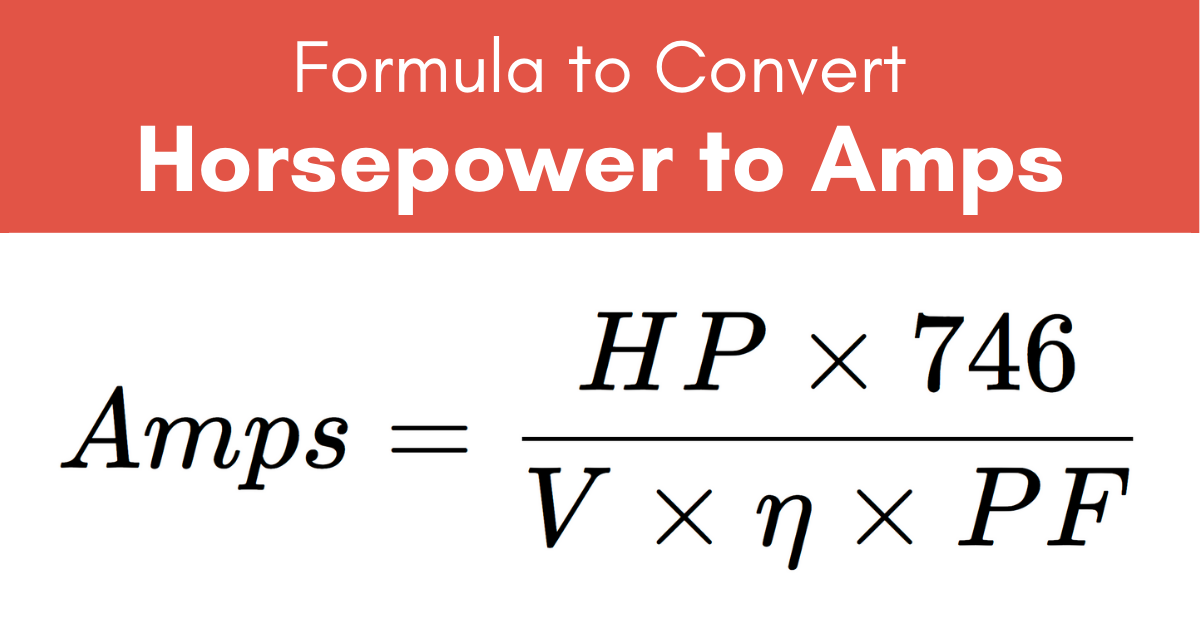# Horsepower to Amps Calculator

Enter horsepower, volts, and motor efficiency below to convert to amps.

HP
volts
%

## Current:

Amps
Learn how we calculated this below

## How to Convert Horsepower to Amps

Electric horsepower (HP) is a measure of electric power equal to 746 watts (W). Horsepower is commonly used to state the output power of an electric motor.

You can convert horsepower to amps of input current in a few simple steps, or using this simple formula which uses the output power of the motor, efficiency of the motor, RMS voltage input and power factor.

I(A) = P(HP) × 746 / V(V) × η × PF

Thus, the current I in amps is equal to the power P in horsepower times 746, divided by the product of the voltage V, efficiency η, and power factor PF. Continue reading to see how this formula was derived in three basic steps.

### Step One: Convert Horsepower to Watts

The first step to converting the motor’s output HP to amps is to convert HP to watts. Since 1 HP is equal to 746 W, simply multiply the horsepower by 746 for this conversion.

P(W) = P(HP) × 746

### Step Two: Account for Efficiency

Since motors are not 100% efficient, the amount of input power needed to achieve the power output is actually higher depending on the efficiency. To find this, divide the horsepower by the efficiency η in decimal form.

The efficiency is given as a fraction between 0 (0%) and 1 (100%).

PIN(W) = POUT(W) / η

We can substitute in the previous equation to convert the output power value to be specified in horsepower.

PIN(W) = POUT(HP) × 746 / η

### Step Three: Convert to Amps

After the power has been converted to watts, use the Watt’s Law power formula to find current in amps using AC RMS Voltage and power factor.

I(A) = P(W) / (V(V) × PF))

Substituting the equations for power above, the final formula to convert horsepower to amps is:

I(A) = P(HP) × 746 / V(V) × η × PF

So, the current I in amps is equal to power P in horsepower times 746, divided by the voltage V times the efficiency η times the power factor PF.For example, let’s find the current draw in amps of a 1-horsepower motor that runs at 120 volts with a 90% efficiency, and the power factor is 1.

I(A) = 1 HP × 746 / 120 V × .9
I(A) = 746 / 120 V × .9
I(A) = 746 / 108 V
I(A) = 6.9 A

In this example, one horsepower is equal to 6.9 amps.

## Common Horsepower to Amps Conversions

Current measurements in amps for common horsepower values at 120 volts for a motor with 90% efficiency.
Horsepower Amps Voltage Efficiency
0.25 HP 1.73 A 120 V 90%
0.5 HP 3.45 A 120 V 90%
0.75 HP 5.18 A 120 V 90%
1 HP 6.91 A 120 V 90%
1.25 HP 8.63 A 120 V 90%
1.5 HP 10.4 A 120 V 90%
1.75 HP 12.1 A 120 V 90%
2 HP 13.8 A 120 V 90%
2.5 HP 17.3 A 120 V 90%
3 HP 20.7 A 120 V 90%
3.5 HP 24.2 A 120 V 90%
4 HP 27.6 A 120 V 90%
4.5 HP 31.1 A 120 V 90%
5 HP 34.5 A 120 V 90%
10 HP 69.1 A 120 V 90%

Also be sure to check out our amps to horsepower calculator.

## References

1. Miller, C., NFPA's Electrical References, National Fire Protection Association, 2004 , Jones & Bartlett Learning, 67-75. https://www.google.com/books/edition/NFPA_s_Electrical_References/raUyIi7i-asC